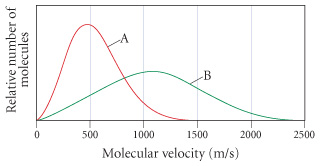# Problem: The following graph shows the distribution of molecular velocities for two different molecules (A and B) at the same temperature. Which molecule would have the higher rate of effusion? a) A b) B c) not enough information to determine

###### FREE Expert Solution

rate of effusion → proportional to molecular velocity###### Problem Details

The following graph shows the distribution of molecular velocities for two different molecules (A and B) at the same temperature. Which molecule would have the higher rate of effusion?a) A

b) B

c) not enough information to determine